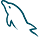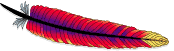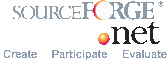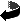Powered by© October 2006 Lorentz JÄNTSCHIUp This is a job type application. It works mainly in console. The web interface is only for displaying the results. It is about use of graph polynomials such as counting polynomial and characteristic polynomial in molecular design.
Demo version (only polynomials calculation): GP.php?job=cX. Files: cX. MathCad file for ChP: cX.mcd.
• ChP - Characteristic polynomial
• CDi - Counting polynomial on Distance Matrix
• CMx - Counting polynomial on Maximal Fragments Matrix
• CcM - Counting polynomial on Complementary of Maximal Fragments Matrix
• CSz - Counting polynomial on (Unsymmetrical) Szeged Matrix
• CCf - Counting polynomial on (Unsymmetrical) Cluj Fragmental Matrix

If still you want to make a computation on your own molecule, please use the following: